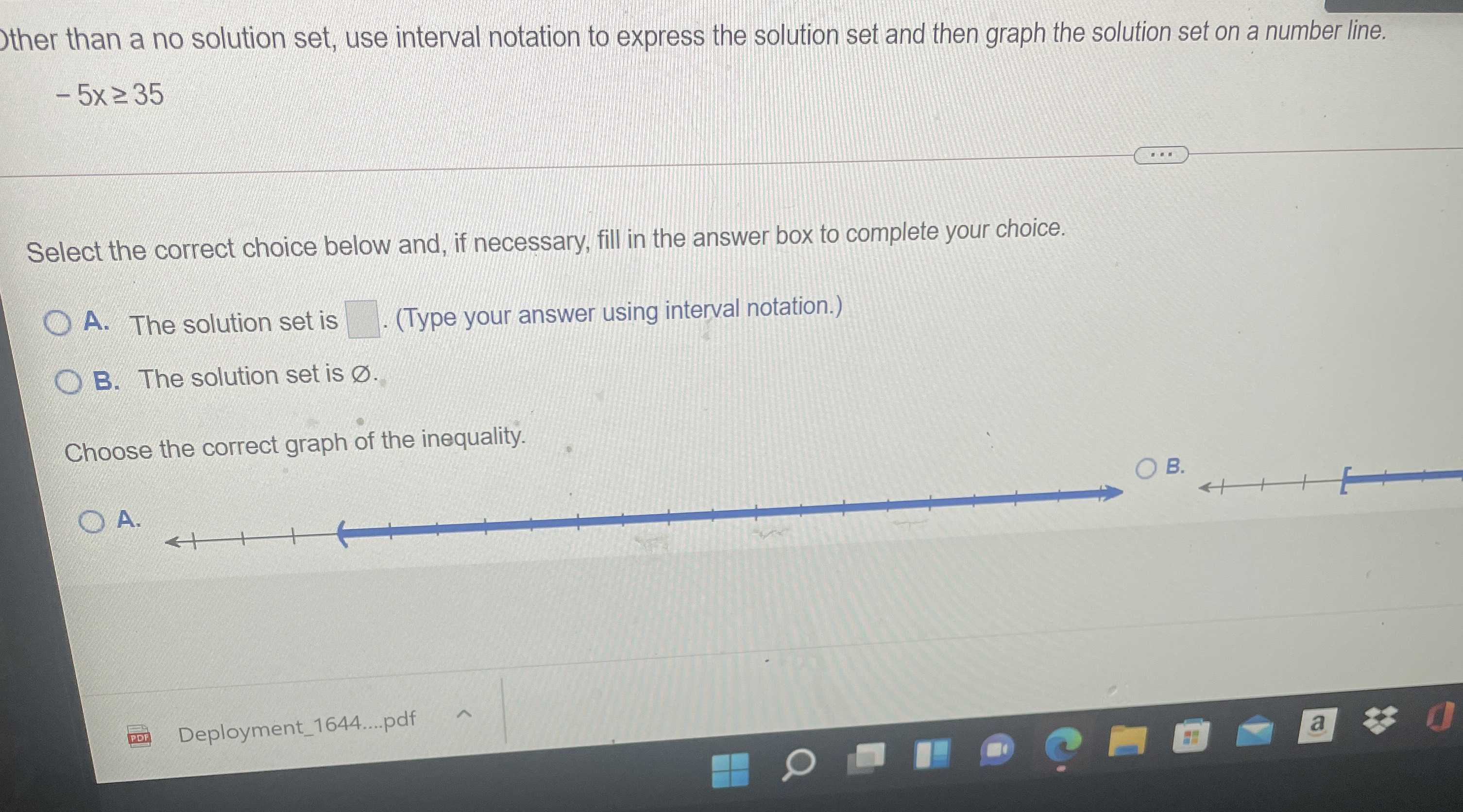### Still have math questions?

Algebra
QuestionOther than a no solution set, use interval notation to express the solution set and then graph the solution set on a number line.

$$- 5 x \geq 35$$

Select the correct choice below and, if necessary, fill in the answer box to complete your choice. A. The solution set is . (Type your answer using interval notation.) B. The solution set is $$\varnothing$$ . Choose the correct graph of the inequality.

$$- 5 x \geq 35$$
Solution: $$\quad x \leq - 7$$
Interval Notation: $$\quad ( - \infty , - 7 ] ]$$